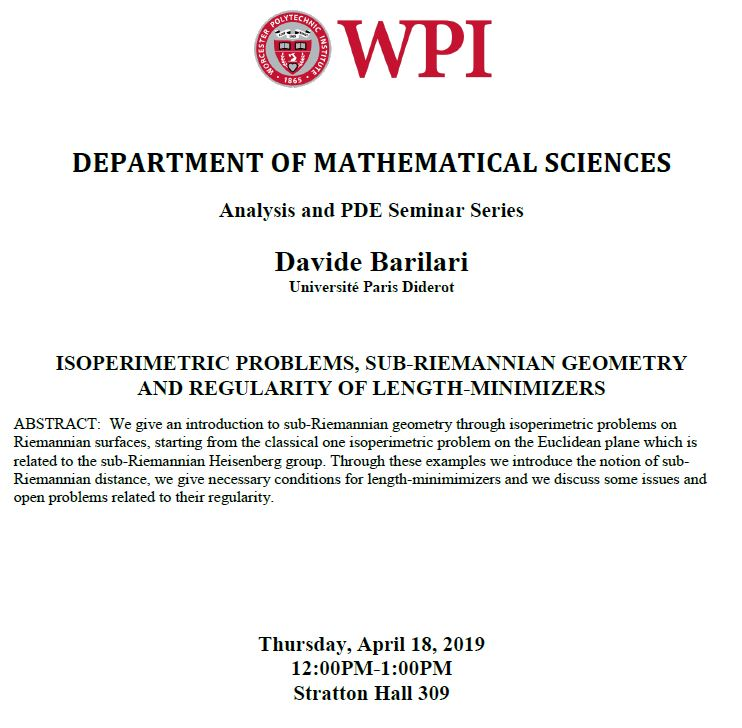# Mathematical Sciences - Analysis and PDE Seminar - Davide Barilari, (Université Paris Diderot) "Isoperimetric problems, sub-Riemannian geometry and regularity of length-minimizers"

## Capture.JPGThursday, April 18, 2019
12:00 pm to 1:00 pm

## Location:

Floor/Room #:
309

Speaker: Davide Barilari, Université Paris Diderot

Title: Isoperimetric problems, sub-Riemannian geometry and regularity of length-minimizers​

Abstract: We give an introduction to sub-Riemannian geometry through isoperimetric problems on Riemannian surfaces, starting from the classical one isoperimetric problem on the Euclidean plane which is related to the sub-Riemannian Heisenberg group. Through these examples we introduce the notion of sub-Riemannian distance, we give necessary conditions for length-minimimizers and we discuss some issues and open problems related to their regularity.

Audience(s):
Attachment: